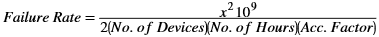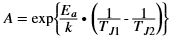# Failure Rate Determination

## Device Reliability Report (UG116)

Document ID
UG116
Release Date
2023-05-17
Revision
10.17 English
The failure rate is typically defined in FIT units. One FIT equals 1 failure per 1 billion device hours. For example, 5 failures expected out of 1 million components operating for 1,000 hours have a failure rate of 5 FIT. The following is the failure rate calculation method:where:

x2 = Chi-squared value at a desired confidence level and (2f + 2) degrees of freedom, where f is the number of failures.

The acceleration factor is calculated using the Arrhenius relationship:where:

E a = Thermal activation energy (0.7 eV is assumed and used in failure rate calculation except EPROM in which 0.58 eV is used).

A = Acceleration factor

k = Boltzmann's constant, 8.617164 x 10-5 eV/K

T J1 = Use junction temperature in Kelvin (K = °C + 273.16)

T J2 = Stress junction temperature in Kelvin (K = °C + 273.16)